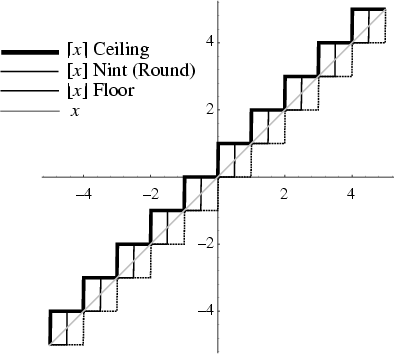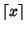## Floor FunctionThe functionis the largest Integer, shown as the dashed curve in the above plot, and also called the Greatest Integer Function. In many computer languages, the floor function is called the Integer Part function and is denoted int(x). The name and symbol for the floor function were coined by K. E. Iverson (Graham et al. 1990).

Unfortunately, in many older and current works (e.g., Shanks 1993, Ribenboim 1996), the symbolis used instead of. Because of the elegant symmetry of the floor function and Ceiling Function symbolsand, and becauseis such a useful symbol when interpreted as an Iverson Bracket, the use ofto denote the floor function should be deprecated. In this work, the symbolis used to denote the nearest integer Nint function since it naturally falls between theandsymbols.

References

Graham, R. L.; Knuth, D. E.; and Patashnik, O. Integer Functions.'' Ch. 3 in Concrete Mathematics: A Foundation for Computer Science. Reading, MA: Addison-Wesley, pp. 67-101, 1990.

Iverson, K. E. A Programming Language. New York: Wiley, p. 12, 1962.

Ribenboim, P. The New Book of Prime Number Records. New York: Springer-Verlag, pp. 180-182, 1996.

Shanks, D. Solved and Unsolved Problems in Number Theory, 4th ed. New York: Chelsea, p. 14, 1993.

Spanier, J. and Oldham, K. B. The Integer-Value Int() and Fractional-Value frac() Functions.'' Ch. 9 in An Atlas of Functions. Washington, DC: Hemisphere, pp. 71-78, 1987.